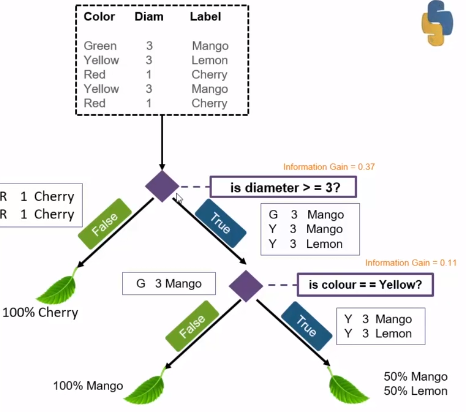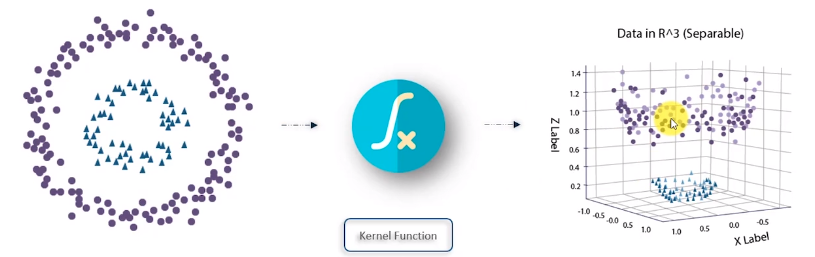# Supervised Learning: Glance of the powerful Classification algorithms

`Types of Classification Algorithms1. Logistic Regression2. Decision Tree3. Random Forest4. K Nearest Neighbour5. Naive byes6. SVM7. XGBOOST`
1. Logistic Regression
• Graphical representation of all possible solutions to a decision made by some conditions
• Can be easily explained and conceptualizedGini Impurity of the leaf node is zero
1. Root Node — It represents the entire population or sample, and this further gets divided into two or more homogenous sets.
2. Leaf Node — Node cannot be segregated further in nodes (Gini index =0 at a leaf node)
3. Splitting — Dividing the root node/sub node into different parts based on some condition.
4. Subtree — Intermediate step of splitting a tree
5. Pruning — Removing unwanted branches from the tree
6. Parent/Child node — Root node is the parent node and all other nodes branching from it is called child node
1. Entropy — Defines randomness in the data. It is a metric which measures the impurity. The first step to solve the decision tree problem.
2. Information Gain — The information gain is the decrease in entropy after a dataset is split based on an attribute. Constructing a decision tree is all about finding an attribute that returns the highest information gain.
3. Gini Index- The measure of impurity (or purity) used in building the decision tree is Gini index
4. Reduction in variance- Algorithm used for continuous target variables (regression problem). The split with lower variance is selected as the criteria to split the population
`Formula of EntropyE(S) = -P(Yes)log2 P(Yes)-P(No) log2 P(no)When P(yes)=P(no)=0.5 , Equal no. of yes and noE(S) = -0.5log2 0.5 - 0.5 log2 0.5E(S) =-0.5(log2 0.5 - log2 0.5) =1E(S) = -P(Yes)log2 P(Yes)When P(yes)=1 , Only yes in sample spaceE(S) = 1 log2 1 =0Similarily for P(No)=1 Only no in sample spceE(S) = 1 log2 1 =0Information Gain = Entropy(S) -[(Weighted Avg)x Entropy(each feature)]   ; s- total collection`

## The important concept of Confusion Matrix

A confusion matrix is a table that is often used to describe the performance of a classification model (or “classifier”) on a set of test data for which the true values are known.

• Summarizes the count value of correct and incorrect predictions(grouped by class)
• true positives (TP): These are cases in which we predicted yes (they have the disease), and they do have the disease.
• true negatives (TN): We predicted no, and they don’t have the disease.
• false positives (FP): We predicted yes, but they don’t have the disease. (Also known as a “Type I error.”)
• false negatives (FN): We predicted no, but they do have the disease. (Also known as a “Type II error.”)
`from sklearn.metrics import confusion_matrixexpected  = [1,1,0,1,0,1,1,0,0,0]predicted = [1,0,0,1,0,0,1,0,1,1]results = confusion_matrix(expected,predicted)print(results)#it means the system predict 6 times correctly and 2 times incorrectly as 1,0 in predictedo/p:[[3 2] [2 3]]`
• Builds multiple decision trees and merges them to get a more accurate and stable prediction. For prediction takes an average of all decision trees
• Correct decision tree’s characteristics of overfitting the training set. Uses Bagging method — building multiple decision trees by using a random set of dataset
• Called Random because Each decision tree in forest considers a random subset of features while forming questions and have access to only a random set of training data sets, that why it’s robust.

## 4. KNN

• use data and classify new data points based on similarity measures. used in search applications. Seen in Flipkart if you purchase a shirt it recommends products from different categories like pants etc
1. select K =number of nearest neighbor
1. suppose we introduce a new data point (star) and K=3, Using the least distance it finds it has 2 orange points and 1 blue point as its closest three neighbors (k=3) so it will be classified as yellow
2. For K=6, similarly, it has 6 blue points and 2 yellow points it is classified as blue.
• lazy learner — decides on the time of prediction, not intuitive training phase
1. Handle Dataset
2. Similarity — Calculate the distance between two data measures
3. Neighbors — Locate K most similar data instances
4. Response — Generate a response from a set of data instance (prediction)
5. Accuracy — summarizing the accuracy of prediction
• probabilistic method of machine learning, based on Bayes Theorem
• Assumption — The presence of a particular feature in a class is not related to the presence of any other feature.
• Bayes theorem shows the realtion between a conditional probablity and its reverse form
`EX: A event : Patient has lung disease , Past data says 10% of patients had lung disease, P(A) =0.1B event: Patient smokes, 5% are smoker ,P(B)=0.05you know that among the patients having lung disease , 7% are smokerP(B/A) =0.07so by bayes thereomP(A|B) =(0.07 *0.1)/0.05`

## 6. Support Vector Machine

• Support vector machine is a supervised machine learning algorithm which classifies data based on its features
• SVM separates data using hyperplanes. There are infinite ways of drawing hyperplanes to separate data, to select the best fit we use support vectors
• Support vectors are the two nearest data points to the hyperplane
• The optimal hyper-plane would have the maximum distance between the support vectors
• The distance between the support vectors is known as margin
• svn uses Kernel function to transform 2D non-linear data to higher dimensionsKernels Fn — Polynomial, Gaussian, Gaussian Radial Basis and Laplace RBF Kernel

## 7. Random Forest

This is an ensemble method , combine several base models in order to produce one optimal predictive model

## 8.XGBOOST

Implementation: on Iris Data set

--

--